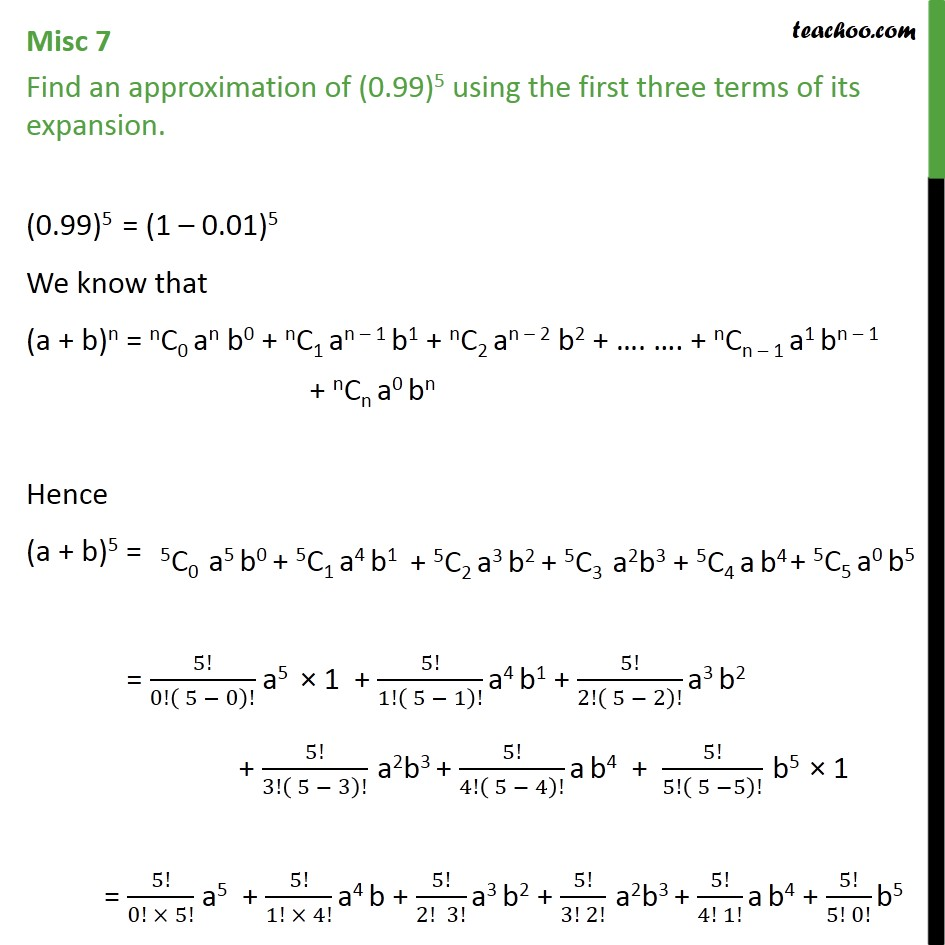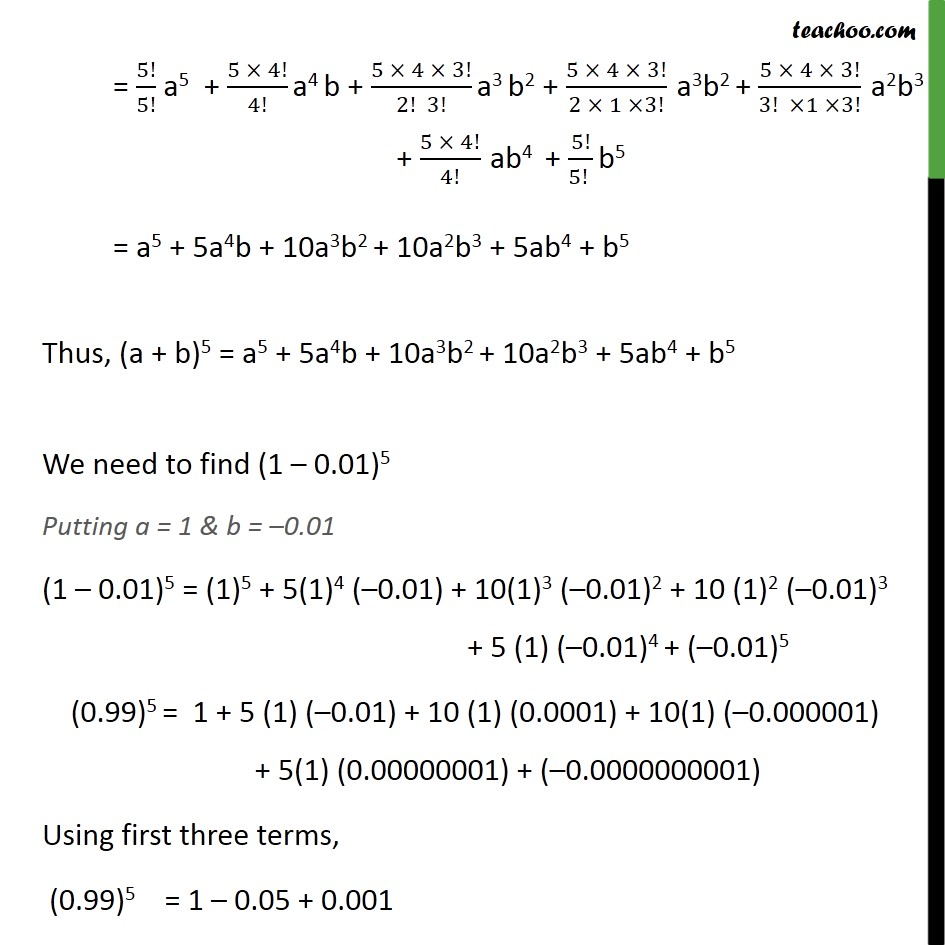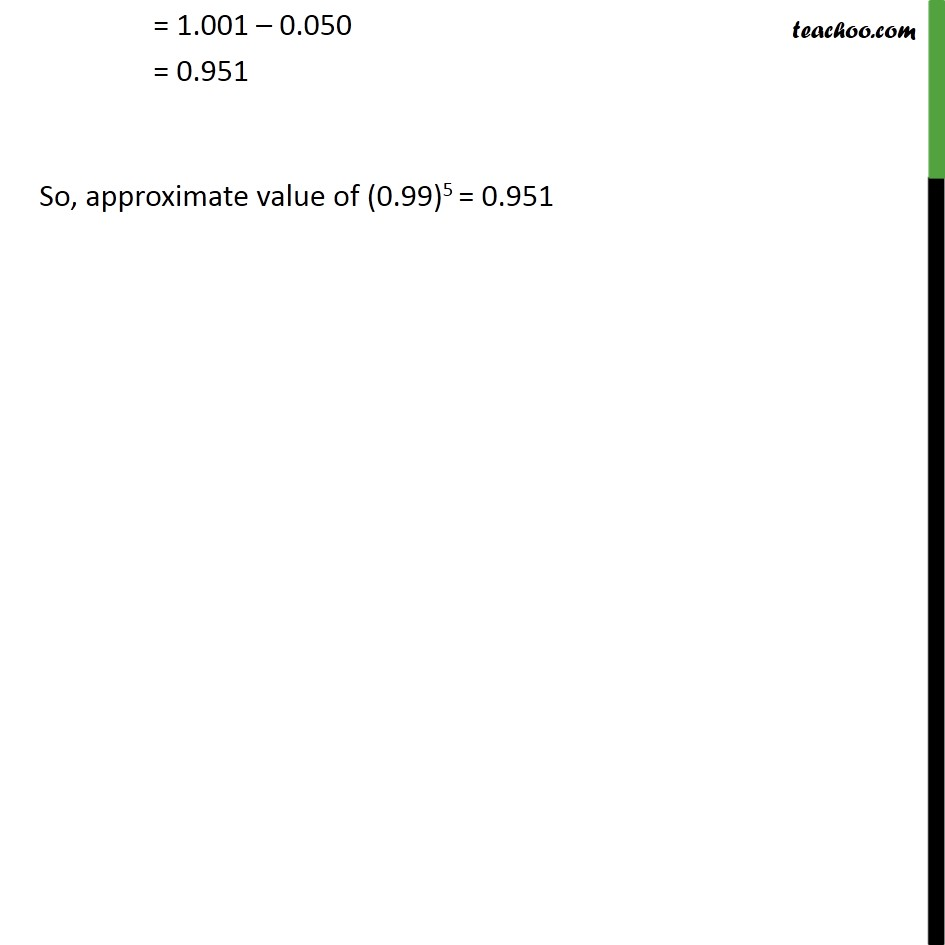1. Chapter 8 Class 11 Binomial Theorem
2. Serial order wise
3. Miscellaneous

Transcript

Misc 7 Find an approximation of (0.99)5 using the first three terms of its expansion. (0.99)5 = (1 – 0.01)5 We know that (a + b)n = nC0 an b0 + nC1 an – 1 b1 + nC2 an – 2 b2 + …. …. + nCn – 1 a1 bn – 1 + nCn a0 bn Hence (a + b)5 = = ﷐5!﷮0!﷐ 5 − 0﷯!﷯ a5 × 1 + ﷐5!﷮1!﷐ 5 − 1﷯!﷯ a4 b1 + ﷐5!﷮2!﷐ 5 − 2﷯!﷯ a3 b2 + ﷐5!﷮3!﷐ 5 − 3﷯!﷯ a2b3 + ﷐5!﷮4!﷐ 5 − 4﷯!﷯ a b4 + ﷐5!﷮5!﷐ 5 −5﷯!﷯ b5 × 1 = ﷐5!﷮0! × 5!﷯ a5 + ﷐5!﷮1! × 4!﷯ a4 b + ﷐5!﷮2! 3!﷯ a3 b2 + ﷐5!﷮3! 2!﷯ a2b3 + ﷐5!﷮4! 1!﷯ a b4 + ﷐5!﷮5! 0!﷯ b5 = ﷐5!﷮5!﷯ a5 + ﷐5 × 4!﷮4!﷯ a4 b + ﷐5 × 4 × 3!﷮2! 3!﷯ a3 b2 + ﷐5 × 4 × 3!﷮2 × 1 ×3!﷯ a3b2 + ﷐5 × 4 × 3!﷮3! ×1 ×3!﷯ a2b3 + ﷐5 × 4!﷮4!﷯ ab4 + ﷐5!﷮5! ﷯ b5 = a5 + 5a4b + 10a3b2 + 10a2b3 + 5ab4 + b5 Thus, (a + b)5 = a5 + 5a4b + 10a3b2 + 10a2b3 + 5ab4 + b5 We need to find (1 – 0.01)5 Putting a = 1 & b = –0.01 (1 – 0.01)5 = (1)5 + 5(1)4 (–0.01) + 10(1)3 (–0.01)2 + 10 (1)2 (–0.01)3 + 5 (1) (–0.01)4 + (–0.01)5 (0.99)5 = 1 + 5 (1) (–0.01) + 10 (1) (0.0001) + 10(1) (–0.000001) + 5(1) (0.00000001) + (–0.0000000001) Using first three terms, (0.99)5 = 1 – 0.05 + 0.001 = 1.001 – 0.050 = 0.951 So, approximate value of (0.99)5 = 0.951

Miscellaneous